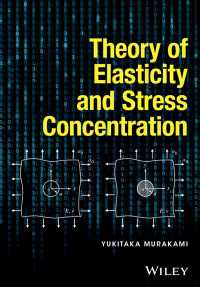## 電子書籍詳細Theory of Elasticity and Stress Concentration

1

Murakami, Yukitaka

Wiley 2016/09
472p.

ISBN: 9781119274094
eISBN: 9781119274100
KNPID: EY00105750

ファイルフォーマット:
ファイルサイズ:
デバイス:ご購入を希望される方は、下のリンクをクリックしてください。

### Full Description

Theory of Elasticity and Stress Concentration

Yukitaka Murakami, Kyushu University, Japan

A comprehensive guide to elasticity and stress concentration

Theory of Elasticity and Stress Concentration comprehensively covers elasticity and stress concentration and demonstrates how to apply the theory to practical engineering problems. The book presents a new approach to the topic without the need for complicated mathematics, and the principles and meaning of stress concentration are covered without reliance on numerical analysis.

The book consists of two parts: Part I - Theory of Elasticity and Part II - Stress Concentration. Part I treats the theory of elasticity from the viewpoint of helping the reader to comprehend the essence of it. Part II treats the principle and meaning of stress concentration and guides the reader to a better understanding of it. Throughout the book, many useful and interesting applications of the basic new way of thinking are presented and explained.

Key features:

• Unique approach to the topics.
• Encourages the readers to acquire the new way of thinking and engineering judgement.
• Includes examples, problems and solutions.

This book provides essential reading for researchers and practitioners in the structural and mechanical engineering industries.

Part I

Preface for the book

Preface for the part

Chapter 1 Stress

1.1 Stress at the surface of a body

1.1.1 Normal stress

1.1.2 Shear stress

1.2 Stress in the interior of a body

1.3 Two-dimensional (2D) stress state, three-dimensional (3D) stress state and stress transformation

1.3.1 Normal stress

1.3.2 Shear stress

1.3.3 Stress in an arbitary direction

1.3.3.1 Two-dimensional stress transformation

1.3.3.2 Three-dimensional stress transformation

1.3.4 Principal stresses

1.3.4.1 Principal stresses in two-dimensional stress state

1.3.4.2 Principal stresses in three-dimensional stress state

1.3.5 Pricipal shear stresses

Problems of Chapter 1

Chapter 2 Strains

2.1 Strains in two-dimensional problems

2.2 Strains in three-dimensional problems

2.3 Strains in an arbitrary direction

2.3.1 Two-dimensional case

2.3.2 Three-dimensional case

2.4 Principal strains

2.5 Conditions of compatibility

Problems of Chapter 2

Chapter 3 The Relationship between Stresses and Strains: The Generalized Hooke’s law

Problems of Chapter 3

Chapter 4 Equilibrium Equations

Problems of Chapter 4

Chapter 5 Saint Venant’s Principle and Boundary Conditions

5.1 Saint Venant’s Principle

5.2 Boundary conditions

Problems of Chapter 5

Chapter 6 Two-Dimensional Problems

6.1 Plane stress and plane strain

6.2 Basic conditions for exact solutions: Nature of solutions

6.3 Airy’s stress function

6.4 Hollow cylinder

6.5 Stress concentration at a circular hole

6.6 Stress concentration at an elliptical hole

6.7 Stress concentration at a hole in a finite width plate

6.8 Stress concentration at a crack

6.9 Stress field due to a point force applied at the edge of a semi-infinite plate

6.10 Circular disk subjected to a concentrated force

Problems of Chapter 6

Appendix of Chapter 6

Chapter 7 Torsion of a Bar with Uniform Section

7.1 Torsion of cylindrical bars

7.2 Torsion of bars having thin closed section

7.3 Saint Venant’s torsion problems

7.4 Stress function in torsion

7.5 Membrane analogy: Solution of torsion problems by using the deformation of pressurized membrane

7.6 Torsion of bars having thin unclosed section

7.7 Comparison of torsional rigidity between a bar with an open section and a bar with a closed section

Problems of Chapter 7

Chapter 8 Energy Principles

8.1 Strain energy

8.2 Uniqueness of the solutions of elasticity problems

8.3 Principle of the virtual work

8.4 Principle of the minimum potential energy

8.5 Castigliano’s theorem

8.6 The reciprocal theorem

Problems of Chapter 8

Chapter 9 The Finite Elemenet Method, FEM

9.1 FEM for one-dimensional problems

9.2 Analaysis of plane stress problems by the finite element method

9.2.1 Approximation of 2D plane stress problems by a set of triangular elements

9.2.2 Relationship between stress and strain in plane stress problemm

9.2.3 Stiffness matrix of a triangular plate element

9.2.4 Stiffness matrix of the total structure

9.2.5 Expression of boundary conditions and basic knowledge for element meshing

Problems of Chapter 9

Chapter 10 Bending of Plates

10.1 Simple examples of plate bending

10.2 General problems of plate bending

10.3 Transformation of bending moment and torsional moment

10.4 Differential equations for a plate subjected to loads on the surface and their applications

10.5 Boundary conditions in plate bending problems

10.6 Polar coordinate expression of the quantities of plate bending

10.7 Stress concentration in plate bending problems

10.8 Bending of a circular plate

Problems of Chapter 10

Chapter 11 Deformation and Stress in Cylindrical Shells

11.1 Basic equations

11.2 Various problems of cylindrical shells

Problems of Chapter 11

Chapter 12 Thermal Stress

12.1 Therrmal stress in a rectangular plate – Simple examples of thermal stress

12.2 Thermal stress in a circular plate

12.3 Thermal stress in a cylinder

Problems of Chapter 12

Chapter 13 Contact Stress

13.1 Two-dimensional contact stress

13.2 Three-dimensional contact stress

Problems of Chapter 13

Appendix

Appendix 1 Rule of direction cosines

Appendix 2 Green’s theorem and Gauss’ divergence theorem

Answers and Hints for the Problems